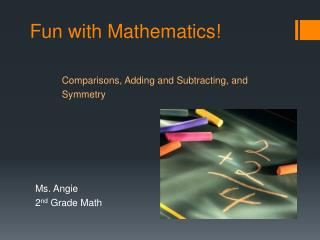DownloadDownload PresentationFun with Mathematics! Comparisons, Adding and Subtracting, and Symmetry

# Fun with Mathematics! Comparisons, Adding and Subtracting, and Symmetry

Télécharger la présentation## Fun with Mathematics! Comparisons, Adding and Subtracting, and Symmetry

- - - - - - - - - - - - - - - - - - - - - - - - - - - E N D - - - - - - - - - - - - - - - - - - - - - - - - - - -
##### Presentation Transcript

1. Fun with Mathematics!Comparisons, Adding and Subtracting, and Symmetry Ms. Angie 2nd Grade Math

2. Learning Objectives! • You will be able to: • Compare whole numbers up to 999 • Add and Subtract number combinations up to 20 • Identify symmetry Based on South Carolina Standards: 2-2.4 2-2.8 2-4.2

3. Comparisons • What is a comparison? • Compare two numbers to find out which is bigger, smaller, or the same • 21 is smaller than 37 • 393 is bigger than 112 • 863 is the same as 863

4. Add numbers together 7 + 8 = ________ 10 + 1 = ________ 11 + 9 = ________ Subtracting Numbers 20 – 7 = ________ 8 – 2 = ________ 16 – 7 = ________ 15 13 11 6 Adding and Subtracting 20 9 When you ADD numbers you combine them When you SUBTRACT numbers you take them away

5. Symmetry Click me!